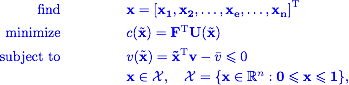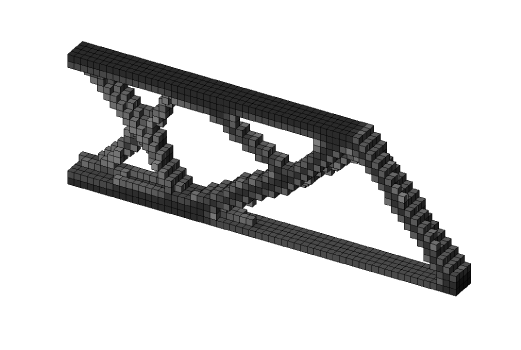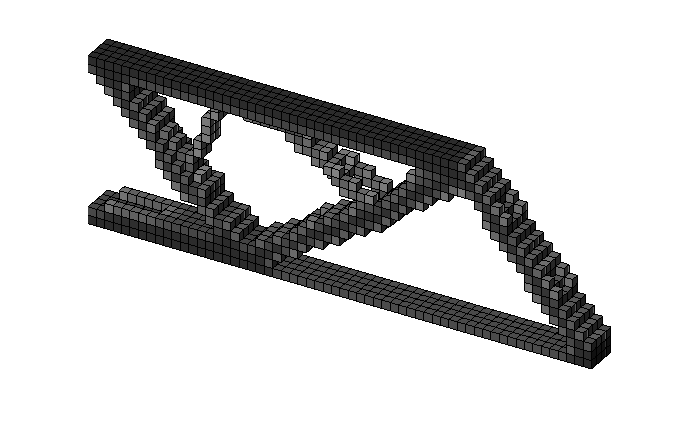# Errata - An efficient 3D topology optimization code written in Matlab

This post lists all known errors in K. Liu and A. Tovar, “An efficient 3D topology optimization code written in Matlab”, Struct Multidisc Optim, doi:10.1007/s00158-014-1107-x.

### Section 4.1 Minimum compliance

Line under (18):

where the physical densities $\tilde{\mathbf{x}} = \tilde{\mathbf{x}} \left( \tilde{\mathbf{x}} \right)$ are defined by (3),

should be:

where the physical densities $\tilde{\mathbf{x}} = \tilde{\mathbf{x}} \left( \mathbf{x} \right)$ are defined by (3),

### Section 4.3 Heat conduction

The equilibrium condition for heat transfer in finite element formulation is described by

where $\mathbf{U}(\mathbf{k}_i^0)$ now donates the finite element global nodal temperature vector,

should be:

The equilibrium condition for heat transfer in finite element formulation is described by

where $\mathbf{U}(\tilde{\mathbf{x}})$ now donates the finite element global nodal temperature vector,

(33):  The optimization problem for heat conduction isshould be:### Section 6.1.3 Active and passive elements

Fig.7 bottom topology optimized beamshould be:for more details see: active and passive elements tutorial

### Section 6.1.4 Alternative filters

Fig. 8 Topology optimized design used a mesh with $30 \times 10 \times 2$ elements. Left optimized design using density filter, middle left optimized design using density filter, middle right optimized design using density filter and gray scale filter, and right optimized design using sensitivity filter and gray scale filter

should be written:

Fig. 8 Topology optimized design used a mesh with $60 \times 20 \times 4$ elements. Left optimized design using density filter, middle left optimized design using sensitivity filter, middle right optimized design using density filter and gray scale filter, and right optimized design using sensitivity filter and gray scale filter for more details see: Sensitivity filter, Grayscale filter

### Section 6.2 Compliant mechanism synthesis

Ud = U(:,1);
U  = U(:,2);
ce = reshape(sum((U(edofMat)*KE).*Ud(edofMat),2),[nely,nelx,nelz]);
c  = U(dout,1);
dc = penal*(E0-Emin)*xPhys.^(penal-1).*ce;


should be written:

U1 = U(:,1);
U2 = U(:,2);
ce = reshape(sum((U1(edofMat)*KE).*U2(edofMat),2),[nely,nelx,nelz]);
c  = U(dout,1);
dc = penal*(E0-Emin)*xPhys.^(penal-1).*ce;


for more details see: compliant mechanisms synthesis tutorial

### Appendix A: Symbolic expression of $\mathbf{k}^0_i$

$% $

On 4th column and 2nd row should be $\mathbf{k}_3^\text{T}$ instead of $\mathbf{k}_4^\text{T}$:

Thanks to Sebastian Białkowski, Technical University of Lodz in Poland

### Appendix B: Derivation of the Hessian Matrix

The reduced Hessian matrix presented in the paper is inaccurate. Please see Evgrafov, A. Struct Multidisc Optim (2014) 50: 1197. https://doi.org/10.1007/s00158-014-1204-x for the detailed discussion.

### Appendix C: Line 103 k

The factor of the $k$ matrix should be $1/144$ instead of $1/72$. Line 103 should be written as:

k = 1/144 * A' * [1; nu];


This change has been applied to top3d.m